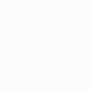# Tag: error bar

2k views

Hello,
I am working on a chart that contains a number of StackedColumnRenderableSeries. Each series has a unique StackedGroupId set so all of the columns display side-by-side (“grouped” mode). I have attached a screenshot of what the chart currently looks like.

The Y-values in the chart are each an average of several collected data values, so I would like to be able to include the standard deviations as error bars drawn over each column, with the center at the top of the column and the high and low values set to center+SD and center-SD.

What would be the best approach to do this? The first thing that comes to mind for me is to create a new class StackedColumnRenderableSeriesWithErrorBars which derives from StackedColumnRenderableSeries, override InternalDraw, and add the drawing of error bars to InternalDraw after calling base.InternalDraw. Of course this would only work as long as the DataSeries is an HlcDataSeries instead of an XyDataSeries.

I also considered an approach that involved overriding/modifying FastErrorBarsRenderableSeries to essentially add a “grouped” mode, but I’m not sure there’s a good way to add that.

I believe the StackedColumnRenderableSeriesWithErrorBars approach will work, but I want to make sure I’m not missing a simpler approach that I could take instead. Any help would be greatly appreciated.

Thank you!

UPDATE: I have it working to the point where it draws the error bars, but the X coordinate is always in the middle of the group, rather than being centered in the middle of the column. I assume I need to make use of the Wrapper (IStackedColumnsWrapper) in the StackedColumnRenderableSeries to shift the X coordinate, but I’m having a little trouble figuring out exactly how. Here is what I have thus far:

``````public class StackedColumnRenderableSeriesWithErrorBars : StackedColumnRenderableSeries
{
public bool ShowErrorBars { get; set; } = false;

protected override void InternalDraw(IRenderContext2D renderContext, IRenderPassData renderPassData)
{
base.InternalDraw(renderContext, renderPassData);

// Now that the main drawing of the StackedColumnRenderableSeries is done, draw the error bars (if applicable).
if (ShowErrorBars)
{
// The resampled data for this render pass
// Don't try to draw error bars if the PointSeries isn't an HlcPointSeries.
if (renderPassData.PointSeries is not HlcPointSeries dataPointSeries)
{
return;
}
ICoordinateCalculator<double> xCalc = renderPassData.XCoordinateCalculator;
ICoordinateCalculator<double> yCalc = renderPassData.YCoordinateCalculator;

// Iterate over the point series
for (int i = 0; i < dataPointSeries.Count; i++)
{
// Get the values
double x = dataPointSeries.XValues[i];
double high = dataPointSeries.HighValues[i];
double low = dataPointSeries.LowValues[i];

// Transform to coordinate
double xCoord = xCalc.GetCoordinate(x);
double highCoord = yCalc.GetCoordinate(high);
double lowCoord = yCalc.GetCoordinate(low);

// TODO: Use Wrapper to transform the X coordinate based on where in the group this column is.

// TODO: Set cap width based on column width
double capWidth = 10;

using IPen2D pen = renderContext.CreatePen(Colors.Black, true, 2);
// Draw vertical line
renderContext.DrawLine(pen, new Point(xCoord, highCoord), new Point(xCoord, lowCoord));
// Draw top cap
renderContext.DrawLine(pen, new Point(xCoord - (capWidth / 2), highCoord), new Point(xCoord + (capWidth / 2), highCoord));
// Draw bottom cap
renderContext.DrawLine(pen, new Point(xCoord - (capWidth / 2), lowCoord), new Point(xCoord + (capWidth / 2), lowCoord));
}
}
}
}
``````
1 vote
2k views

I’m building a chart where I’m plotting range vs doppler and the range has some error in it. There is a need for me to show the upper and lower bounds of that error. The issue I’m having is that for this particular chart the range is the x value. I have an estimated range but I need to show that there is some error in it. How do I make the error bar horizontal? I saw in the Android SDK there is a method to set the error bar direction. Is there something I can do in WPF to change the orientation of the error bar?

Showing 2 results

## Try SciChart Today

Start a trial and discover why we are the choice
of demanding developers worldwide